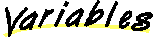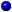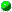# Class IndexNode

```java.lang.Object
|
+----IndexNode
```

public class IndexNode
extends Object
An index node for a simulated file system.

Author:
Ray Ontko

##INDEX_NODE_SIZE
Size of each index node in bytes.MAX_DIRECT_BLOCKS
Maximum number of direct blocks in an index node.MAX_FILE_BLOCKS
Maximum number of blocks in a file.

##IndexNode()
Creates an index node.

##copy(IndexNode)getAtime()getBlockAddress(int)
Gets the address corresponding to the specified sequential block of the file.getCtime()getGid()getMode()
Gets the mode for this IndexNode.getMtime()getNlink()
Get the number of links for this IndexNode.getSize()
Gets the size for this IndexNode.getUid()main(String[])
A test driver for IndexNode.read(byte[], int)
Reads the contents of an index node from a byte array.setAtime(int)setBlockAddress(int, int)
Sets the address corresponding to the specified sequential block of the file.setCtime(int)setGid(short)setMode(short)
Sets the mode for this IndexNode.setMtime(int)setNlink(short)
Set the number of links for this IndedNode.setSize(int)
Sets the size for this IndexNode.setUid(short)toString()
Converts an index node into a printable string.write(byte[], int)
Writes the contents of an index node to a byte array.

##INDEX_NODE_SIZE
``` public static final int INDEX_NODE_SIZE
```
Size of each index node in bytes.MAX_DIRECT_BLOCKS
``` public static final int MAX_DIRECT_BLOCKS
```
Maximum number of direct blocks in an index node.MAX_FILE_BLOCKS
``` public static final int MAX_FILE_BLOCKS
```
Maximum number of blocks in a file. If indirect, doubleIndirect, or tripleIndirect blocks are implemented, this number will need to be increased.

##IndexNode
``` public IndexNode()
```
Creates an index node.

##setMode
``` public void setMode(short newMode)
```
Sets the mode for this IndexNode. This is the file type and file protection information.getMode
``` public short getMode()
```
Gets the mode for this IndexNode. This is the file type and file protection information.setNlink
``` public void setNlink(short newNlink)
```
Set the number of links for this IndedNode.

Parameters:getNlink
``` public short getNlink()
```
Get the number of links for this IndexNode.

Returns:setUid
``` public void setUid(short newUid)
```getUid
``` public short getUid()
```getGid
``` public short getGid()
```setGid
``` public void setGid(short newGid)
```setSize
``` public void setSize(int newSize)
```
Sets the size for this IndexNode. This is the number of bytes in the file.getSize
``` public int getSize()
```
Gets the size for this IndexNode. This is the number of bytes in the file.getBlockAddress
``` public int getBlockAddress(int block) throws Exception
```
Gets the address corresponding to the specified sequential block of the file.

Parameters:
block - the sequential block number
Returns:
the address of the block, a number between zero and one less than the number of blocks in the file system
Throws: Exception
if the block number is invalidsetBlockAddress
``` public void setBlockAddress(int block,
```
Sets the address corresponding to the specified sequential block of the file.

Parameters:
block - the sequential block number
address - the address of the block, a number between zero and one less than the number of blocks in the file system
Throws: Exception
if the block number is invalidsetAtime
``` public void setAtime(int newAtime)
```getAtime
``` public int getAtime()
```setMtime
``` public void setMtime(int newMtime)
```getMtime
``` public int getMtime()
```setCtime
``` public void setCtime(int newCtime)
```getCtime
``` public int getCtime()
```write
``` public void write(byte buffer[],
int offset)
```
Writes the contents of an index node to a byte array. This is used to copy the bytes which correspond to the disk image of the index node onto a block buffer so that they may be written to the file system.

Parameters:
buffer - the buffer to which bytes should be written
offset - the offset from the beginning of the buffer at which bytes should be writtenread
``` public void read(byte buffer[],
int offset)
```
Reads the contents of an index node from a byte array. This is used to copy the bytes which correspond to the disk image of the index node from a block buffer that has been read from the file system.

Parameters:
buffer - the buffer from which bytes should be read
offset - the offset from the beginning of the buffer at which bytes should be readtoString
``` public String toString()
```
Converts an index node into a printable string.

Returns:
the printable string
Overrides:
toString in class Objectcopy
``` public void copy(IndexNode indexNode)
```main
``` public static void main(String args[]) throws Exception
```
A test driver for IndexNode.

Throws: Exception
any exception which may occur